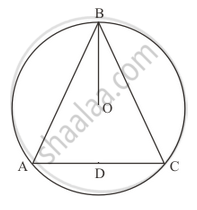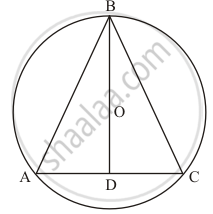Advertisement Remove all ads

# In the Given Figure, O Is the Centre of the Circle, Bo Is the Bisector of ∠Abc. Show That Ab = Bc. - Mathematics

Answer in Brief

In the given figure, O is the centre of the circle, BO is the bisector of ∠ABC. Show that AB = BC.Advertisement Remove all ads

#### Solution

It is given that,∠ABC is on circumference of circle BD is passing through centre.Construction: Join A and C to form AC and extend BO to D such that BD be the perpendicular bisector of AC.

Now in  $\bigtriangleup BDA \text{ and } \bigtriangleup BDC$ we have

AD = CD           (BD is the perpendicular bisector)

So ,

$\angle BDA = \angle BDC = 90°$
BD = BD  (Common)
$\bigtriangleup BDA \cong \bigtriangleup BDC \left( \text{ SAS congruency criterion } \right)$
Hence AB =BC   (by cpct)
Is there an error in this question or solution?
Advertisement Remove all ads

#### APPEARS IN

RD Sharma Mathematics for Class 9
Chapter 15 Circles
Exercise 15.4 | Q 5 | Page 73
Advertisement Remove all ads

#### Video TutorialsVIEW ALL 

Advertisement Remove all ads
Share
Notifications

View all notifications

Forgot password?
Course